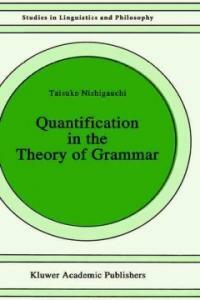> 상세정보

# 상세정보## Quantification in the theory of grammar (5회 대출)

자료유형
단행본
개인저자
Nishigauchi, Taisuke.
서명 / 저자사항
Quantification in the theory of grammar / Taisuke Nishigauchi.
발행사항
Dordrecht :   Kluwer Academic Pub.,   c1990.
형태사항
xiii, 239 p. ; 23 cm.
총서사항
Studies in linguistics and philosophy ;v. 37
ISBN
0792306430
일반주기
Includes index
서지주기
Bibliography: p. 230-233
일반주제명
Grammar, Comparative and general --Quantifiers. Government --binding theory (Linguistics)
 000 00707camuuu200217 4500 001 000000231386 005 19961010133151.0 008 910205s1990 ne b 00110 eng 020 ▼a 0792306430 049 0 ▼l 412682605 090 ▼a 415 ▼b N724q 100 1 0 ▼a Nishigauchi, Taisuke. 245 1 0 ▼a Quantification in the theory of grammar / ▼c Taisuke Nishigauchi. 260 0 ▼a Dordrecht : ▼b Kluwer Academic Pub., ▼c c1990. 300 ▼a xiii, 239 p. ; ▼c 23 cm. 440 ▼a Studies in linguistics and philosophy ; ▼v v. 37 500 ▼a Includes index 504 ▼a Bibliography: p. 230-233 650 0 ▼a Grammar, Comparative and general ▼x Quantifiers. 650 ▼a Government ▼x binding theory (Linguistics)

### 소장정보

No. 소장처 청구기호 등록번호 도서상태 반납예정일 예약 서비스
No. 1 소장처 청구기호 415 N724q 등록번호 412682605 도서상태 대출가능 반납예정일 예약 서비스

### 컨텐츠정보

#### 목차

```
CONTENTS
PREFACE = xi
LIST OF ABBREVIATIONS = xiii
CHAPTER Ⅰ: INTRODUCTION = 1
1. WH-phrases as quantificational expressions = 1
2. Locality = 6
3. Quantificational force = 12
CHAPTER Ⅱ: SUBJACENCY AND LOGICAL FORM = 14
1. Introduction = 14
2. WH-Movement in Japanese = 17
3. Subjacency = 25
3.1. The WH-Island Condition effect = 26
3.2. Discourse-oriented factors = 33
3.2.1. Focus = 33
3.3. The Complex NP Constraint = 40
4. ECP vs. Pied-piping = 43
4.1. The ECP account = 43
4.2. Arguments for the pied-piping analysis = 48
4.2.2. The scope problem =58
4.2.3. Weak Crossover = 63
4.2.4. Complement structure = 67
4.2.5. More on Weak Crossover = 73
CHAPTER Ⅲ: THE PIED-PIPING MECHANISM = 75
1. Percolation = 75
2. German relative clauses = 81
3. Restrictions on percolation = 83
3.1. The 'Specificity Condition' effect = 83
3.2. Why: Categorial identity = 88
3.3. Arguments for categorial identity = 93
4. Quantifier vs. Sentential operator = 97
4.1. Overview = 97
4.2. Scope of naze and dooiu-riyuu-de = 100
4.3. Multiple Questions = 104
4.4. Prospects = 109
5. Government and unselective binding = 110
6. Concluding remarks = 112
CHAPTER Ⅳ: CONSTRUING WH = 116
1. 'Indeterminate pronominals' = 116
1.1. The problem = 116
1.2. Some semantics = 123
2. Unselective binding = 128
2.1. Indefinite NPs = 128
2.2. Inherent binding vs. External binding = 130
2.3. Properties of the binding relations = 132
2.3.1. Quantificational force = 133
2.3.2. Selectiveness = 135
2.3.3. Scopal interaction = 137
2.3.4. Locality = 141
2.4. Two types of binding = 145
3. Unselective binding involving WH = 146
4. The movement analysis = 152
4.1. Movement and unselective binding = 152
4.2. More movement = 157
5. Adverbs of quantification = 165
6. Scope interactions and QR = 172
7. Concluding remarks = 176
CHAPTER Ⅴ: THE CASE FROM ENGLISH : THE NO MATTER CONCESSIVE CLAUSE = 177
1. Problems = 177
2. No Matter and WH..Ever constructions = 179
3. Donkey sentences = 180
4. No Matter as unselective binder = 181
4.1. Government = 183
4.2. The WH-Island Condition = 188
4.3. 'Connectedness' and indirect binding = 189
5. Concluding remarks = 197
CHAPTER Ⅵ: THE DONKEY PROBLEM IN JAPANESE = 202
1. Weak Crossover = 202
2. Donkey sentences in Japanese = 204
3. Indirect binding = 208
4. Restrictions on indirect binding = 211
4.1. C-command = 211
4.2. The WH-Island Condition effect = 213
4.3. Apparent violations of Subjacency = 215
4.4. Subject-object asymmetry? = 218
5. Concluding remarks = 226
BIBLIOGRAPHY = 230
INDEX = 234

```